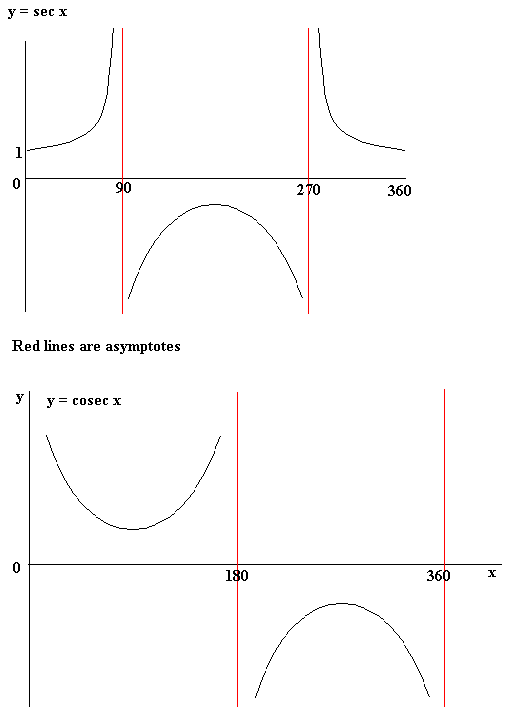## Sec, Cosec and Cot

Secant, cosecant and cotangent, almost always written as sec, cosec and cot are trigonometric functions like sin, cos and tan.

sec x  =    1
cos x

cosec x =    1
sin x
cot x =      1       =   cos x
tan x         sin x

Note, sec x is not the same as cos-1x (sometimes written as arccos x).

Remember, you cannot divide by zero and so these definitions are only valid when the denominators are not zero.

Example

If sec x = 2, cos x = ½, x = 60º

Graphs of sec x and cosec x